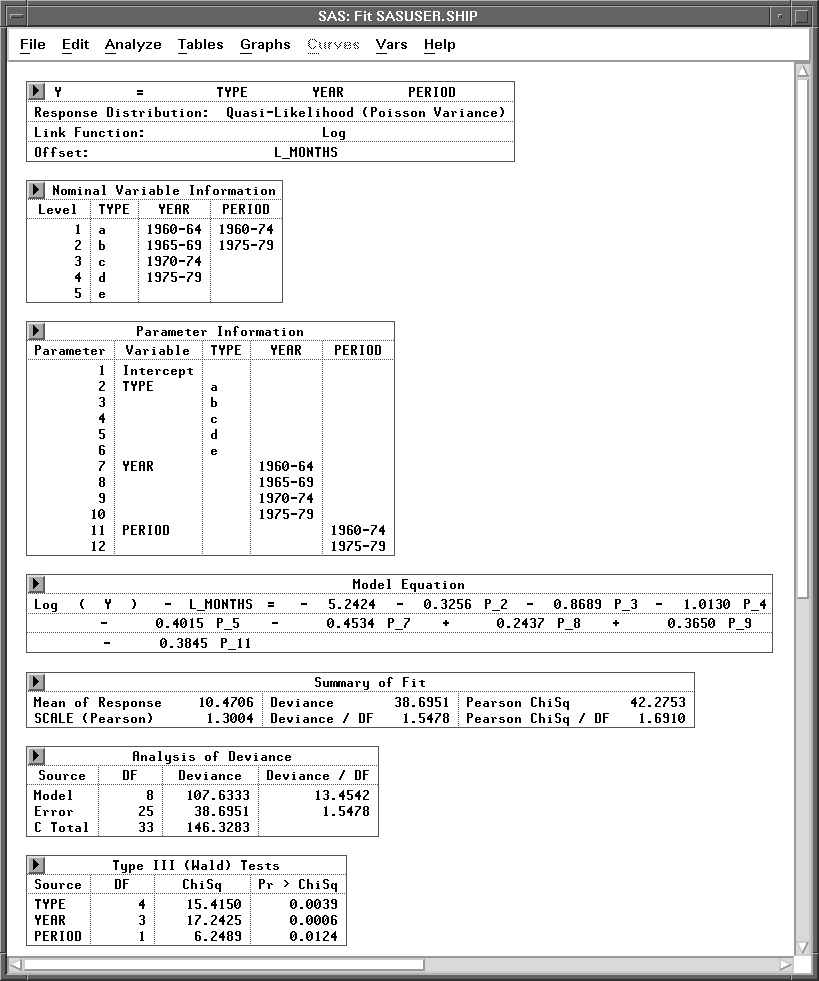Previous Page Next Page
 Poisson Regression

# Poisson Regression

In Chapter 16, "Logistic Regression," you examined logistic regression as an example of a generalized linear model.

In this chapter, you will examine another example of a generalized linear model, Poisson regression. You can choose Analyze:Fit ( Y X ) to carry out a Poisson regression analysis when the response variable represents counts. You can use the fit variables and methods dialogs to specify this generalized linear model.Figure 17.1: Poisson Regression Analysis

#### References

 Previous Page Next Page Top of Page